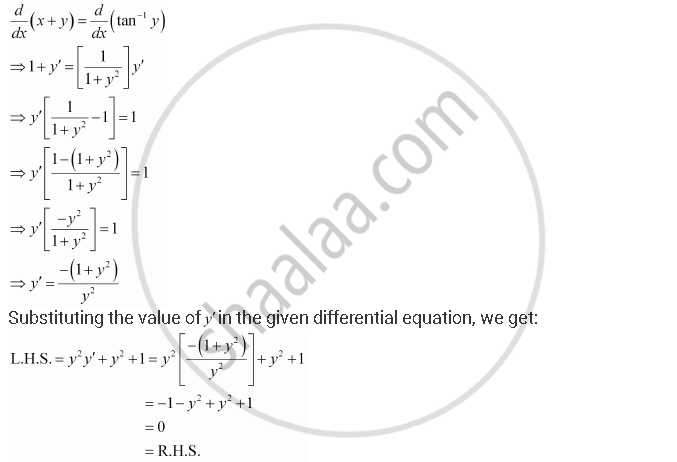Share

# Verify that the Given Functions (Explicit Or Implicit) is a Solution of the Corresponding Differential Equation X + Y = Tan–1y : Y2 Y′ + Y2 + 1 = 0 - CBSE (Science) Class 12 - Mathematics

ConceptGeneral and Particular Solutions of a Differential Equation

#### Question

verify that the given functions (explicit or implicit) is a solution of the corresponding differential equation

x + y = tan–1y   :   y2 y′ + y2 + 1 = 0

#### Solution

x + y = tan–1y

Differentiating both sides of this equation with respect to x, we get:Hence, the given function is the solution of the corresponding differential equation.

Is there an error in this question or solution?

#### APPEARS IN

NCERT Solution for Mathematics Textbook for Class 12 (2018 to Current)
Chapter 9: Differential Equations
Q: 9 | Page no. 385

#### Video TutorialsVIEW ALL 

Solution Verify that the Given Functions (Explicit Or Implicit) is a Solution of the Corresponding Differential Equation X + Y = Tan–1y : Y2 Y′ + Y2 + 1 = 0 Concept: General and Particular Solutions of a Differential Equation.
S